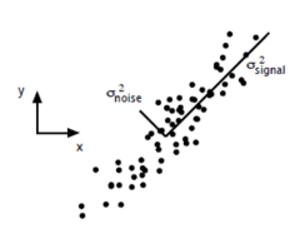# 第一部分：线性变换与特征值和特征向量之间的关系

## 线性变换

(1) 对任意$\alpha,\beta \in V$，有$\sigma(\alpha + \beta) = \sigma(\alpha) + \sigma(\beta)$
(2) 对任意$\alpha\in V$$\lambda \in R$，有$\sigma(\lambda \alpha) = \lambda \sigma(\alpha)$

$\begin{bmatrix} 1 & 0 \\ 0 & 1 \end{bmatrix}\alpha = \alpha$

## 特征值和特征向量的定义

$Aα = λα$

## 对角阵的特征值和特征变量

### 例1

$\begin{vmatrix} \lambda-1 & 0 \\ 0 & \lambda-1 \end{vmatrix} = 0$

$(A-\lambda E) X= 0$

### 例2

$\begin{vmatrix} \lambda-1 & 0 \\ 0 & \lambda-3 \end{vmatrix} = 0$

$\lambda_1=1$带入方程
$(A-\lambda E) X= 0$

$\lambda_2=3$带入方程
$(A-\lambda E) X= 0$

## 实对称矩阵的特征值和特征向量的性质

### 例2

Spectral Theorem:一个线性变换（用矩阵乘法表示）可表示为它的所有的特征向量的一个线性组合，其中的线性系数就是每一个向量对应的特征值。写成公式就是：
$T(v) = \lambda_1(v1\cdot v)v_1 + \lambda_2(v2\cdot v)v_2 + ...$

1. 实对称矩阵的特征值都是实数，特征向量都是实向量。
2. 实对称矩阵的不同特征值对应的特征向量是正交的。
3. n阶实对称矩阵A的一个k重的特征值$\lambda$必有k个线性无关的特征向量。或者说必有秩$r(\lambda E-A)=n-k$

## 相似矩阵

$P^{-1}AP = B$

# 第二部分：数据矩阵与特征值和特征向量之间的关系

## 特征值分解

$A = Q\Sigma Q^{-1}$

## 奇异值分解(SVD)

$A = U\Sigma V^{T}$

n的矩阵，则得到的U是一个mm的方阵（里面的向量是正交的，称为左奇异向量），$\Sigma$是一个mn的矩阵（除了对角线上的元素外都是0，对角线上的元素称为奇异值），$V^T$是一个n*n的方阵（里面的向量是正交的，称为右奇异向量）。

$(A^TA)V_i = \lambda_iv_i$

$\sigma_i = \sqrt{\lambda_i}$
$u_i = \frac{1}{\sigma_i}Av_i$

$A_{m*n}\approx U_{m*r}\Sigma_{r*r}V^T_{r*n}$

# 第三部分：主成分分析（PCA-Principle Component Analysis）（一般来说，方差大的方向是信号的方向，方差小的方向是噪声的方向，我们在数据挖掘中或者数字信号处理中，往往要提高信号与噪声的比例，也就是信噪比。对上图来说，如果我们只保留signal方向的数据，也可以对原数据进行不错的近似了。这也就是“降噪”的原理。）
PCA的全部工作简单点说，就是在原始的空间中顺序地找一组相互正交的坐标轴，第一个轴是使得方差最大的，第二个轴是在与第一个轴正交的平面中使得方差最大的，第三个轴是在与第1、2个轴正交的平面中方差最大的，这样假设在N维空间中，我们可以找到N个这样的坐标轴，我们取前r个去近似这个空间，就可以提取数据的前r个“主成分”了。

### 补充知识：矩阵与向量的乘积的几何意义

$A = \begin{bmatrix} a_{11} & a_{12} &... & a_{1n} \\ a_{21} & a_{22} &... & a_{2n} \\ ...\\ a_{m1} & a_{m2} &... & a_{mn} \end{bmatrix}$

$A = \begin{bmatrix} \alpha_1\\ \alpha_2 \\ ...\\ \alpha_m \end{bmatrix}$

$\alpha_1 = (a_{11}, a_{12},... , a_{1n} )$
$\alpha_2 = (a_{21}, a_{22},... , a_{2n} )$
$...$
$\alpha_m = (a_{m1}, a_{m2},... , a_{mn} )$

$A\cdot u = \begin{bmatrix} \alpha_1\\ \alpha_2 \\ ...\\ \alpha_m \end{bmatrix}\cdot u = \begin{bmatrix} \alpha_1 u\\ \alpha_2 u\\ ...\\ \alpha_m u \end{bmatrix}$

### PCA的推导

$A = \begin{bmatrix} a_{11} & a_{12} &... & a_{1n} \\ a_{21} & a_{22} &... & a_{2n} \\ ...\\ a_{m1} & a_{m2} &... & a_{mn} \end{bmatrix}$

$Var(Au) = (Au-E)^T(Au-E)=(Au)^T(Au)=u^TA^TAu$

$J(u) = u^TA^TAu$

$L(u) = u^TA^TAu - \lambda(u^Tu-1)$

$\frac{dL(u)}{du}=A^TAu-\lambda u$

$A^TAu=\lambda u$